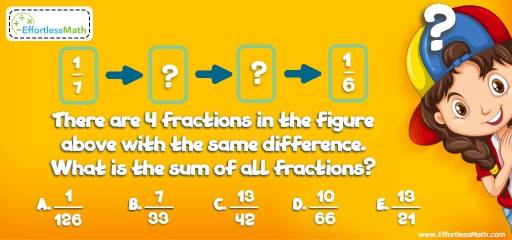# Intelligent Math Puzzle – Challenge 85

Sharing this great and fun math and critical thinking puzzle with students is the best way to get them thinking mathematically.## Challenge:

There are 4 fractions in the figure above with the same difference. What is the sum of all fractions?

A-$$\frac{1}{126}$$

B- $$\frac{7}{33}$$

C- $$\frac{13}{42}$$

D- $$\frac{10}{66}$$

E- $$\frac{13}{21}$$

### The Absolute Best Book to challenge your Smart Student!

There are four fractions with the same difference. First, we need to find the missing fractions.
The difference of $$\frac{1}{7}$$ and $$\frac{1}{6}$$ is:
$$\frac{1}{6} – \frac{1}{7}/7 = \frac{7-6}{42} = \frac{1}{42}$$
The difference of $$\frac{1}{7}$$ and the first fraction is:
$$\frac{1}{42} ÷3 = \frac{1}{126}$$
The first missing fraction is:
$$\frac{1}{7} + \frac{1}{126}= \frac{18+1}{126} = \frac{19}{126}$$
The second missing fraction is:
$$\frac{19}{126} + \frac{1}{126}= \frac{20}{126}$$
The sum of all fractions equals:
$$\frac{1}{7} + \frac{19}{126}+ \frac{20}{126} + \frac{1}{6} = \frac{18}{126}+ \frac{19}{126} + \frac{20}{126} + \frac{21}{126} = \frac{78}{126} = \frac{13}{21}$$

### What people say about "Intelligent Math Puzzle – Challenge 85 - Effortless Math: We Help Students Learn to LOVE Mathematics"?

No one replied yet.

X
30% OFF

Limited time only!

Save Over 30%

SAVE $5 It was$16.99 now it is \$11.99